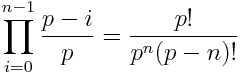# Random number sequence overlap

Mike Croucher asked the following question on his blog. Suppose you draw M sequences of random numbers of length N from a random number generator. What is the probability that they will overlap?

Assumes your random number generator is a cyclical list of p unique integers. Each draw picks a random starting point in the cycle and chooses N consecutive values. We are particularly interested in the case M = 104, N = 109, and p = 219937 – 1 (the period of the Mersenne Twister).

We will simplify the problem by over-estimating the probability of overlap. The over-estimated probability will still be extremely small.

Assume all the draws are independent. This greatly increases the probability of repetition because now there is some chance that there are repetitions within each draw. That wasn’t possible before, provided N < p.

The probability of no repetitions in n = MN draws isWe can under-estimate this probability by replacing every term in the product with the smallest term. (By under-estimating the probability of no repetitions, we’re over-estimating the probability of at least one repetition.)When n = MN = 1013 and p = 219937 – 1, the last expression above is approximately 1 – 1026/219937. That says the (over-estimated) probability of repetition is 1026/219937 or about 2×10-5976, thousands of orders of magnitude smaller than the chances of, say, drawing a royal flush.

Whenever you have such mind-bogglingly small probabilities, some probability outside your model has to be more important than the probability computed with the model. For example, we could question the assumption that the starting points are random and independent. Or we could question whether the random number generator was written correctly, or that the compiler correctly compiled the program, or that the operating system correctly ran the program, etc.

## 6 thoughts on “Random number sequence overlap”

1. Jim Steele

Nice article, but all instances of ‘random’ should be replaced by ‘pseudo-random’. I admit that I spend an inordinate amount of time thinking about the difference. I know that computer science says that a Turning machine with a random number generator is supposed to have no more ‘computational power’ than one without random number generation. This would seem to imply the definition of computation is insufficient for tasks such as “generate an arbitrarily long one-time-pad cryptographic key” or “produce an algorithm for playing repeated games of rock-paper-scissors.”

2. Could you please define the term “overlap” as it’s used here? Specifically, I’m curious about how much overlap is required to constitute overlap. If less than 100%, can the portions of overlap be non-consecutive? Thanks.

3. I would define overlap here as the random number generator returning to the identical internal state. This does not mean just returning the same output twice. Two different internal states could return the same output occasionally. But if the internal states were ever identical, every output from that point on would be the same between the two sequences.

4. John,

Thanks for taking the time to respond with a clarification of the term “overlap”. I’m a bit surprised by your definition, though, especially given the nature of this probability question. Perhaps I’m still not understanding the problem.

Given the deterministic way a pseudo-random number generator, like the Mersenne Twister, works your definition of overlap appears simply to be concerned with matching the internal state (i.e. the seed value) rather than comparing the actual output of the PRNG. Given that, it’s hard for me to understand why N, the length of the sequences, is even relevant to the question. As you said, if the seed, or internal state, of the PRNG is the same, the resulting output, of any length, will be the same. It also seems that the probability of overlap (i.e. matching the internal state, or seed value) is simply 1 in the period of the PRNG, which is 1 / 2^19937 − 1 for the MT.

My guess is that the original question had more to do with the probability of collisions in the output rather than matching the internal state. That would necessitate a different definition for overlap. But again, perhaps I’m not fully understanding the question.

Thanks

5. Here’s why the internal state and not the output is what’s important. Suppose you’re using a PRNG to generate coin flips. First you get a head, then a tail. The next output will duplicate one you’ve already seen. But that doesn’t mean the PRNG is stuck in a cycle.

6. Correct, and if you were to see 100, or even 1000, heads in a row, that still doesn’t mean that the PRNG is necessarily stuck in a cycle. My point was that I believe the probability of matching the internal state is based upon the period of the PRNG, not the size of M or N. Unless of course you’re looking at this as an extension of the birthday problem, in which case you’re not matching internal state. In that case, I believe the probability would increase as M increases, or N decreases.

Sorry to keep at this, I just find the subject interesting. Hopefully my questions aren’t too tiresome. Best.